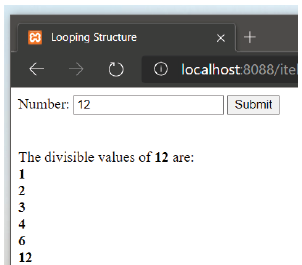# PHP divisible values

How to create a PHP script that will print the corresponding divisible values from a given number inputted. There shall only be one input-box used. Like the sample below:I assume this is school homework. So you won’t be wanting someone to do the job for you, will you, or there would be no learning.

Tell us your thoughts. How far have you got?

1 Like

What’s the definition of a divisible value?
If you have a number X, how many values do you need to check for divisibility?
What is true of a number that is divisible by 6, that you would already know by the time you got to 6?

(that last one’s just for fancy versions that have to handle super large numbers, but isnt probably needed for your homework.)

It only takes a few lines of code to make but can bog down with timeout errors if you enter too large amounts. I think an important step when first learning about forms is to print out `\$_POST` so you get a clear picture of what is being sent when you submit the form, so I would start by making your form and printing out `\$_POST` to test your form, then you can figure out what to do with it.

``````echo "<pre>";
print_r(\$_POST);
echo "</pre>";
``````

As the OP hasn’t returned I will give my thoughts for a bit of a topic closer. As you are looking for numbers that will go into the given number, they would have to be numbers “less than or equal to” the given number. The Comparison Operator for “less than or equal to” would be `<=` so to write this equation out where \$n is the number being tested against the POST number it would be like this `\$n<=\$_POST['number']`. To check all numbers between 1 and the POST number you would set a variable to 1 i.e. `\$n=1;` and then increment this variable within a loop by using `++` after the variable i.e. `\$n++` so the value of `\$n` on each loop increases, 1, 2, 3 etc. Using those 3 bits of code for `\$n` you can then write a `for()` loop, which will say `\$n=1;` and while `\$n<=\$_POST['number']` is TRUE `\$n++`.

``````for(\$n=1;\$n<=\$_POST['number'];\$n++):

endfor;
``````

Adding a line to echo `\$n` will show all numbers as `\$n` is incremented.

``````for(\$n=1;\$n<=\$_POST['number'];\$n++):
echo \$n."<br />";
endfor;
``````

As you want only “Divisible Values” shown, you would need to define what that is. A quick example will help explain what we are looking for. If the input number is 5 and we are looping through numbers 1 - 5, the values of 1 and 5 would of course both be Divisible Values but what about 2, 3 or 4 and what makes them NOT Divisible Values? The number 2 goes into 5 twice with a remainder of 1. The number 3 goes into 5 once with a remainder of 2 and the number 4 goes into 5 once with a remainder of 1. So a “Divisible Value” would have a remainder of 0. Thankfully there is a modulus operator `%` which will return the remainder between the 2 numbers given. Using the example numbers above we would have remainders like this.

1 % 5 = 0
2 % 5 = 1
3 % 5 = 2
4 % 5 = 1
5 % 5 = 0

We have already established that we want a remainder of `0` so using the modulus operator we would write an IF condition using the numbers within the FOR loop looking for the remainder value of 0 and wrap this condition around the echo line.

``````if(\$_POST['number'] % \$n == 0):
echo \$n."<br />";
endif;
``````

When testing the code at this point you might see a number of errors like undefined index etc, and you wouldn’t want to run any code based on values that don’t exist. You can also run into issues when the value is zero so it is best to check that the value is NOT EMPTY and wrap your code in this IF condition. Note: This code is below the form.

``````if(\$_SERVER["REQUEST_METHOD"] == "POST" && !empty(\$_POST['number'])):
//code
endif;
``````

As your example image has a Value shown in the input AFTER POST you could define a variable that can be echoed on the page regardless of form submission.

``````\$number = (!empty(\$_POST['number']) ? \$_POST['number'] : '');
``````

This can be placed above the form and `\$number` echoed as a value on the number input.

``````<input type="text" name="number" value="<?php echo \$number;?>" />
``````

The example also shows the number echoed in a line of text so you could use it here as well.

``````echo 'The divisible values of <b>'.\$number.'</b> are: </br></br>';
``````

All together it is just a few lines of code.

``````<?php
\$number = (!empty(\$_POST['number']) ? \$_POST['number'] : '');
?>
<form action="" method="post">
<table border=0>
<tr>
<td>Number:</td>
<td><input type="text" name="number" value="<?php echo \$number;?>" /></td>
<td><input type="submit" value="Submit" /></td>
</tr>
</table>
</form>
<?php
if(\$_SERVER["REQUEST_METHOD"] == "POST" && !empty(\$_POST['number'])):
echo 'The divisible values of <b>'.\$number.'</b> are: </br></br>';
for(\$n=1;\$n<=\$_POST['number'];\$n++):
if(\$_POST['number'] % \$n == 0):
echo \$n."<br />";
endif;
endfor;
endif;
?>
``````

Couple of thoughts, Drummin:

You can speed up your search by only looking until `sqrt(target)` ; for any number `n` found this way, add two numbers to your pool; `n` and `target/n` (target/n must, by definition of n % target being 0, be an integer).

You will then need to `unique` your result, as a square number would put the square root in twice. There can be no numbers greater than sqrt(target) that have a multiple that hasnt already been covered; the “highest-low” divisor of target will be something multiplied by itself.

2 Likes

This topic was automatically closed 91 days after the last reply. New replies are no longer allowed.